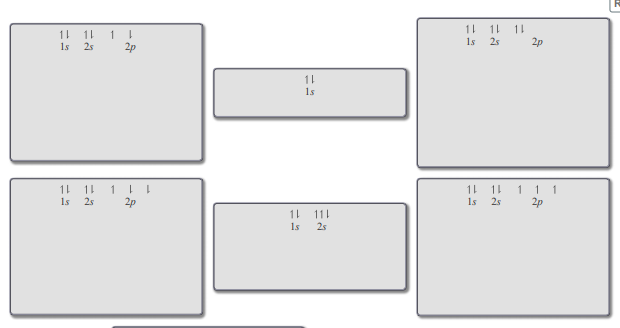# Problem: Part B. Consider that a single box represents an orbital, and an electron is represented as a half arrow. Orbitals of equal energy are grouped together. The orbital diagram will be valid if it follows both Hund's rule and Pauli’s principle. Sort the various orbital diagrams based on whether they are valid, whether they break Hund's rule, or whether they break Pauli's exclusion principle.The electron configuration of an atom describes how the electrons are distributed among the various orbitals of the atom. Watch the animation to learn about the rules that are useful when writing the electron configuration of elements.

###### FREE Expert SolutionView Complete Written Solution
###### Problem Details

Part B. Consider that a single box represents an orbital, and an electron is represented as a half arrow. Orbitals of equal energy are grouped together. The orbital diagram will be valid if it follows both Hund's rule and Pauli’s principle. Sort the various orbital diagrams based on whether they are valid, whether they break Hund's rule, or whether they break Pauli's exclusion principle.The electron configuration of an atom describes how the electrons are distributed among the various orbitals of the atom. Watch the animation to learn about the rules that are useful when writing the electron configuration of elements.